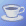#Interactive Real Analysis

Next | Previous | Glossary | Map

## 6.2. Continuous Functions

### Your browser can not handle Java Applets ...

 This graph shows the function f(x) = x4 + 0.5 if x < 1 f(x) = 0.5 x + 1 if 1x < 2 f(x) = cos(x) + 1 if 2x To use the Java applet: Click on Options for control panel Select x by clicking on the x-axis Select a given epsilon Guess delta and press Apply
If for any given epsilon value you can find a positive delta value such that the red area touches the graph inside the green area, then your function is continuous at the given values of x

### Your browser can not handle Java Applets ...

 This graph shows the function f(x) = x2 + 1.3 if x < 1 f(x) = sin(x - 1) + 2 if 1x To use the Java applet: Click on Options for control panel Select x by clicking on the x-axis Select a given epsilon Guess delta and press Apply
If for any given epsilon value you can find a positive delta value such that the red area touches the graph inside the green area, then your function is continuous for x = c
The first function is continuous for all x, while the second function is not continuous for x = 1 and continuous for all other x.Compare with uniform continuity.

Next | Previous | Glossary | Map